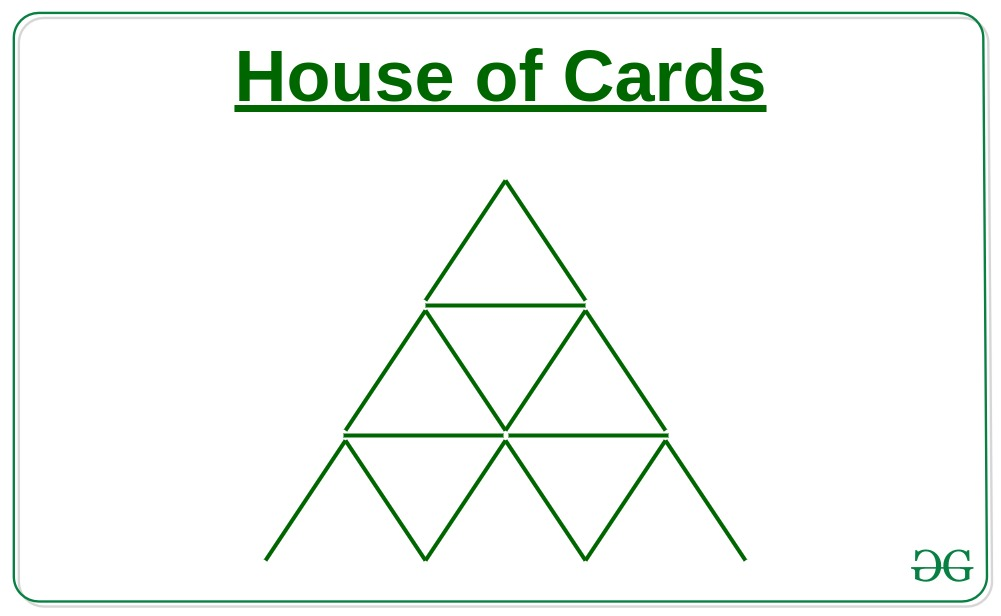# Number of cards needed build a House of Cards of a given level N

• Last Updated : 16 Jun, 2021

Given a number N, the task is to find the number of cards needed to make a House of Cards of N levels.Attention reader! Don’t stop learning now. Get hold of all the important DSA concepts with the DSA Self Paced Course at a student-friendly price and become industry ready.  To complete your preparation from learning a language to DS Algo and many more,  please refer Complete Interview Preparation Course.

In case you wish to attend live classes with experts, please refer DSA Live Classes for Working Professionals and Competitive Programming Live for Students.

Examples:

Input: N = 3
Output: 15
From the above image, it is clear that for the House of Cards for 3 levels 15 cards are needed
Input: N = 2
Output:

Approach:

1. If we observe carefully, then a series will be formed as shown below in which i-th item denotes the number of triangular cards needed to make a pyramid of i levels:

2, 7, 15, 26, 40, 57, 77, 100, 126, 155………and so on.

1.
2. The above series is a method of difference series where differences are in AP as 5, 8, 11, 14……. and so on.

3. Therefore nth term of the series will be:

```nth term = 2 + {5 + 8 + 11 +14 +.....(n-1) terms}
= 2 + (n-1)*(2*5+(n-1-1)*3)/2
= 2 + (n-1)*(10+(n-2)*3)/2
= 2 + (n-1)*(10+3n-6)/2
= 2 + (n-1)*(3n+4)/2
= n*(3*n+1)/2;```
1.
2. Therefore the number of cards needed for building a House of Cards of N levels will be:

``
1.

Below is the implementation of the above approach:

## CPP

 `// C++ implementation of the above approach` `#include ``using` `namespace` `std;` `// Function to find number of cards needed``int` `noOfCards(``int` `n)``{``    ``return` `n * (3 * n + 1) / 2;``}` `// Driver Code``int` `main()``{``    ``int` `n = 3;``    ``cout << noOfCards(n) << ``", "``;``    ``return` `0;``}`

## Java

 `// Java implementation of the above approach``import` `java.lang.*;` `class` `GFG``{``    ``// Function to find number of cards needed``    ``public` `static` `int` `noOfCards(``int` `n)``    ``{``        ``return` `n * (``3` `* n + ``1``) / ``2``;``    ``}``    ` `    ``// Driver Code``    ``public` `static` `void` `main(String args[])``    ``{``        ``int` `n = ``3``;``        ``System.out.print(noOfCards(n));``    ``}``}` `// This code is contributed by shubhamsingh10`

## Python3

 `# Python3 implementation of the above approach` `# Function to find number of cards needed``def` `noOfCards(n):``    ``return` `n ``*` `(``3` `*` `n ``+` `1``) ``/``/` `2` `# Driver Code``n ``=` `3``print``(noOfCards(n))` `# This code is contributed by mohit kumar 29`

## C#

 `// C# implementation of the above approach``using` `System;` `class` `GFG``{``    ``// Function to find number of cards needed``    ``public` `static` `int` `noOfCards(``int` `n)``    ``{``        ``return` `n * (3 * n + 1) / 2;``    ``}``     ` `    ``// Driver Code``    ``public` `static` `void` `Main(String []args)``    ``{``        ``int` `n = 3;``        ``Console.Write(noOfCards(n));``    ``}``}` `// This code is contributed by 29AjayKumar`

## Javascript

 ``
Output:
`15`

Time Complexity: O(1)

My Personal Notes arrow_drop_up